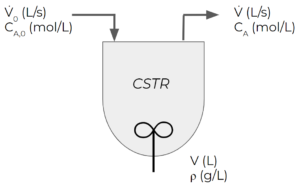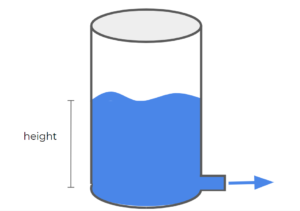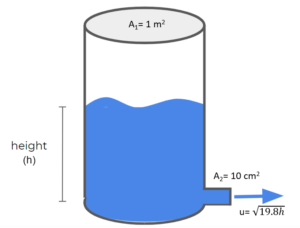# 40 Separable Differential Equations

Learning Objectives

By the end of this section, you should be able to:

Provide initial conditions for well-mixed separable transient single-unit processes.

Solve separable transient balances to find a property of a system at any given time.

## Separable Differential Equations

Separable differential equations are differential equations where the variables can be isolated to one side of the equation. Take the following differential equations:

1 – $\frac{dx}{dy}=(x^{3}+x)*(y-y^{2})$

This equation is separable because you can completely isolate the x and y variables as follows:

$\frac{dx}{x^{3}+x}=dy*(y-y^{2})$

2 – $\frac{dx}{dy}=\frac{x}{x+y}$

This equation is non-separable because you cannot completely isolate the x and y variables:

$dy*(x+y)=dx*x$

These non-separable equations we will discuss later.

Example: Chemical Reactor

Consider a “continuous stirred-tank reactor” (CSTR). CSTRs are reactors with continuous feed and exit streams and some kind of mixer.

• The feed enters at a constant volumetric flowrate of $\dot{V}_{0}$ in L/s
• The volume of the tank is $V$ in L.
• Initially ($t=0$), the tank is filled to $V_i$ in L
• The exit stream flows at a constant rate of $\dot{V}$ in L/s
• We can assume that the density of all streams in the system is constant at $\rho$ in g/LWe want to write a balance for the total (overall) mass in the system under transient conditions.

We start off by writing out the overall balance:

$IN-OUT+GEN-CON=ACC$

Mass is not being consumed or generated, just changed from one substance to another. This means the $GEN$ and $CON$ terms are negligible. We get:

$IN=\dot{V}_{0}*\rho$

$OUT=\dot{V}*\rho$

$ACC=\frac{dM}{dt}=\frac{d(V*\rho)}{dt}=\rho*\frac{dV}{dt}$

The units for the $IN$, $OUT$, and $ACC$ terms are kg/s. Simplifying the balance, we get:

$\dot{V}_{0}*\rho-\dot{V}*\rho = \rho*\frac{dV}{dt}$

Since the densities are all constant, we can cancel them out:

$\dot{V}_{0} - \dot{V} = \frac{dV}{dt}$

Using separation of variables (from calculus), we can integrate both sides. We want to find a given value about our system at a specific final time ($t_{f}$), starting from an initial time (say $t_{0} = 0$). We know the initial volume $V_{i}$ and want to find the final volume $V_{f}$.

$(\dot{V}_{0} - \dot{V})dt = dV$

$\int^{t_{f}}_{t_{0}}(\dot{V}_{0} - \dot{V})dt =\int^{V_{f}}_{V_{i}} dV$

$(\dot{V}_{0} - \dot{V})*(t_{f}-t_{0}) =V_{f}-V_{i}$

$V_{f} = V_{i}+(\dot{V}_{0} - \dot{V})*(t_{f}-t_{0})$

Let’s try substituting in some numbers to this equation. Say the rate of flow in is 5 L/min, and the flow out is 6 L/min with 300 L initially in the tank. How much water remains in the tank after 1 hour? Using the formula we derived, we can find this:

$V_{f} = V_{i}+(\dot{V}_{0} - \dot{V})*(t_{f}-t_{0})$

$V_{f} = 300 L+(5 \frac{L}{min} - 6 \frac{L}{min})*(60 min-0 min) V_{f} = 300 L-60 L V_{f} = 240 L Note, you may say this is obvious and there is no need to derive our equation, and in this case our equation was relatively simple. However, we want to get in the practice of formulating these equations for when things start getting more complicated. In this class, we'll focus on formulating these equations rather than finding the solution. Exercise: Transient Mass Balance Consider pumping liquid from a tank that has one drain line as depicted below:Assume the tank contains only 1 species (A) and the density is constant. What are the relevant terms in the mass balance for this draining tank? Say at time zero, the height of the tank is 5 m, the tank also has a diameter of 2 m. There is a pump at the outlet pumping A out at a rate of 0.1 [latex]m^3/min$. What is the height of the tank at 10 minutes?

### Solution

Step 1: Determine what terms in the general balance are zero or negligible.

$IN - OUT + GEN - CON = ACC$

Since there are no feed streams and no reactions (no mass generated or consumed), the $IN$, $GEN$, and $CON$ terms can be omitted from the balance.

$-OUT = ACC$

Step 2: Write out the mass balance for species A in the system.

$(-\dot{m}_{out,A}) = \frac{dM_{A}}{dt}$

Step 3: Expand the mass terms.

Recall that $Mass=Volume*density$, so we can use that to express both terms in the mass balance:

$(-\dot{V}_{out,A}*\rho) = \rho*\frac{dV_{A}}{dt}$

Since the density is constant, the density terms on both sides cancel each other out

$(-\dot{V}_{out,A}) = \frac{dV_{A}}{dt}$

The volume terms can be further expanded. Recall that the volume of a cylinder $Area*Height$ or $A*h$. In differential terms, $\frac{dV}{dt}=A\frac{dh}{dt} + h\frac{dA}{dt}$. Since the area is constant in this case, this simplifies to: $\frac{dV}{dt} = A\frac{dh}{dt}$.

$(-\dot{V}_{out,A}) = A_{tank}*\frac{dh}{dt}$

$A_{tank} = \frac{\pi}{4}*{D_{tank}}^{2}=\frac{\pi}{4}*{2 m}^{2}=3.14 m^{2}$

Step 4: Solve the integral from t = 0 to t = 10 mins

$(-\dot{V}_{out,A})dt = A_{tank}*dh$

$\int^{t=10 min}_{0 min}(-\dot{V}_{out,A})dt =\int^{h_{final}}_{h_{intial}} A_{tank}*dh$

$\int^{t=10 min}_{0 min}(-0.10\frac{m^3}{min})dt =\int^{h_{final}}_{h_{intial}}3.14m^{2}*dh$

$(-0.1\frac{m^{3}}{min})*t\;\bigg|^{t=10 min}_{0 min} = (3.14m^{2})*h\;\bigg|^{h_{final}}_{5m}$

$(-0.1\frac{m^{3}}{min})*(10min-0min) = (3.14m^{2})*(h_{final}-5m)$

$-1 m^{3}*\frac{1}{3.14m^{2}} = h_{final}-5m$

$-0.32 m = h_{final}-5m$

$(-.32+5) m = h_{final}$

$h_{final}=4.68 m$

Exercise: Transient Mass Balance

Suppose we have a tank with an outlet at the bottom as shown below. Water is the only thing in the tank (we'll call this species A). The water flows out of the tank at a rate of $\sqrt{19.6×h}\;m/s$, where h is the height of water in m at any specific time (note that expression like this for outlet flow come from conservation of energy in a tank with gravity driving flow out of the tank). The tank has a cross-sectional area of $1m^2$ and the outlet pipe has a cross-sectional area $10cm^2$. If the initial height of the water in the tank is 1 m, using a transient mass balance, calculate the height of water in the tank after 5 mins.### Solution

Step 1: Determine what terms in the general balance are zero or negligible.

$IN - OUT + GEN - CON = ACC$

Since there is no inlet stream and no chemical reactions in the tank, there is no $IN$, $GEN$ or $CON$ terms:

$-OUT = ACC$

Step 2: Write out the mass balance for species A in the system.

$(-\dot{m}_{out}) = \frac{dM}{dt}$

Step 3: Express each term using the given quantities:

Replace $\text{Mass}$ by $\text{Volume}×\text{density}$ for both terms:

$(-\dot{V}_{out}×\rho) = \frac{dV×\rho}{dt}$

Because $\rho$ are multiplied by both sides and we assume it is constant throughout the system, we can cancel $\rho$ in this step:

$(-\dot{V}_{out}) = \frac{dV}{dt}$

The volume of fluid flowing out of a pipe at any instant can be calculated using $\dot{V}=u×A$, where u is the instantaneous velocity of the fluid and A is the cross-sectional area of the pipe. We can use this to express the outlet flow. For the accumulation term, it is not beneficial to do this because we don't know the expression for $u$.

For the accumulation term, we can espress dV as $A_{1}×dh$ because we know that $A_{1}$ is constant, and this leaves only one variable (h) for us to solve for in the mass balance:

$-u×A_{2} = \frac{A_{1}dh}{dt}$

We can replace $u$ using $u=\sqrt{19.6×h}$ given in the question. T

$-\sqrt{19.6×h}×A_{2} = \frac{A_{1}dh}{dt}$

Step 4: Solve the integral to find $h_{f}$:

$\int_{t_{0}}^{t_{f}}-\sqrt{19.6×h}×A_{2}\;dt= \int_{h_{0}}^{h_{f}}A_{1}dh$

Separate the equation by moving all terms involving h to one side:

$\int_{t_{0}}^{t_{f}}-\frac{A_{2}}{A_{1}}dt = \int_{h_{0}}^{h_{f}}\frac{1}{\sqrt{19.6×h}}dh$

Replace the variables by the given information: $A_{2}=10cm^2=10×10^{-4}m^2$, $A_{1}=1m^2$, $t_{0}=0s$, $t_{f}=5min=300s$.

\begin{align*}
\int_{0}^{t=300}-\frac{10×10^{-4}}{1} dt&= \int_{h_{0}=1}^{h_{f}}\frac{1}{\sqrt{19.6}}×h^{-\frac{1}{2}}dh \\
-10×10^{-4}× t\;\bigg|_{0}^{t=300}& = \frac{2}{\sqrt{19.6}}×h^{\frac{1}{2}}\;\bigg|_{h_{0}=1}^{h_{f}}\\
-10×10^{-4}×(300-0)& = \frac{2}{\sqrt{19.6}}×h_{f}^{\frac{1}{2}}-\frac{2}{\sqrt{19.6}}×(1)^{\frac{1}{2}}\\
\bigg(\frac{-10×10^{-4}×(300-0)+\frac{2}{\sqrt{19.6}}×(1)^{\frac{1}{2}}}{\frac{2}{\sqrt{19.6}}}\bigg)^2&=h_{f} \\
h_{f}&=0.11m
\end{align*}